# Adjusting for optimism/overfitting in measures of predictive ability using bootstrapping

In a previous post we looked at the area under the ROC curve for assessing the discrimination ability of a fitted logistic regression model. An issue that we ignored there was that we used the same dataset to fit the model (estimate its parameters) and to assess its predictive ability.

A problem with doing this, particularly when the dataset used to fit/train the model is small is that such estimates of predictive ability are optimistic. That is, they will fit the dataset which have been used to estimate the parameters somewhat better than they will fit new data. In some sense, this is because with small datasets the fitted model adapts to chance characteristics of the observed data which won’t occur in future data. A silly example of this would be a linear regression model of a continuous variable Y fitted to a continuous covariate X with just n=2 data points. The fitted line will just be the line connecting the two data points. In this case, the R squared measure will be 1 (100%), suggesting your model has perfect predictive power(!), when of course with new data it would almost certainly not have an R squared of 1.

There are a number of techniques for obtaining predictive ability measures which adjust or correct for this optimism. A simple technique is to split the sample into two parts, using one part to fit the model, and the remainder to assess predictive ability. The problem with this approach is that it is inefficient, since the model is only fitted to a subset of the available data. Better alternatives include cross validation and bootstrapping, among others. For a nice review and investigation into the performance of different approaches in small datasets, see this paper recently published by colleagues in Cambridge.

In this post we’ll look at a bootstrap approach to the problem. For more details on cross validation, I’d recommend looking at chapter 7 of the book “Elements of Statistical Learning”, available for free download from the authors here, or Chapter 5 of the more introductory book “An Introduction to Statistical Learning”, also available for free download (here).

## Bootstrapping in general

Bootstrapping is an amazingly powerful approach for performing certain aspects of statistical inference. In bootstrapping we repeatedly sample from the observed dataset, with replacement, forming a large number (B) of bootstrap datasets, each of the same size as the original data. The idea is that the original observed data takes the place of the population of interest, and the bootstrap samples represent samples from that population.

To use bootstrapping to estimate the standard error of a parameter estimate (for example), we fit our model to the original data, and then also fit the model to each of the B bootstrap sample datasets. Recall that the variance of an estimator is the variance of its value across repeated samples from the population. Since we treat the bootstrap samples as if they are samples from the population, we then use the variability of the point estimates across the B bootstrap datasets as our estimate of variance for the parameter estimate obtained from fitting the model to the original observed data.

## Bootstrapping to allow for optimism

The bootstrap approach we’ll use here is described nicely in a 1996 paper by Frank Harrell and colleagues (freely available here). The approach consists of the following steps, and applies quite generally to measures of predictive ability / explained variation. Here I’ll use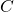(for c-statistic) to denote the measure of predictive accuracy, but it could be something else, like R squared.

1. Fit model to original data, and estimateusing original data based on fitted model. Denote this estimate ofas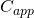.
2. For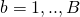:
1. take a bootstrap (with replacement) sample from the original data
2. fit the model to the bootstrap dataset, and estimateusing this fitted model and this bootstrap dataset. Denote the estimate by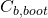3. estimateby applying the fitted model from the bootstrap dataset to the original dataset. Let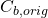denote the estimate
3. Calculate the estimate of optimism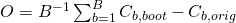4. Calculate the optimism adjusted measure of predictive ability as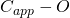This bootstrap approach is very intuitive: usually when we apply a model fitted using a bootstrap dataset to the original data, the predictive accuracy will be lower than the apparent accuracy when evaluating the fitted model using the same data that was used to fit it. We calculate the difference in these predictive abilities for each bootstrap sample, and take the average across many (Harrell et al suggest 100-200 times) bootstrap samples. This estimate of optimism is then subtracted off the naive estimate of predictive ability.

## Implementation in R

The preceding bootstrap approach is implemented in Frank Harrell’s excellent rms package, which is the companion R package to his book, ”Regression Modeling Strategies”. To illustrate, let’s first simulate a simple, small dataset, with a continuous covariate X and a binary outcome Y which depends on X via a logistic regression:

set.seed(63126)
n <- 25
x <- rnorm(n)
pr <- exp(x)/(1+exp(x))
y <- 1*(runif(n) < pr)


Next, we will fit the logistic regression model to the (Y,X) data. Usually we would do this using R's glm function. But in order to use the rms package's validate function, we must use the rms function lrm:

mod <- lrm(y~x, x=TRUE, y=TRUE)


Next we call the rms function validate to perform the bootstrap approach described previously, specifying 1000 bootstrap samples:

validate(mod, B=1000)
index.orig training    test optimism index.corrected    n
Dxy           0.2533   0.2819  0.1905   0.0914          0.1620 1000
R2            0.0651   0.1096  0.0651   0.0445          0.0206 1000
Intercept     0.0000   0.0000  0.2175  -0.2175          0.2175 1000
Slope         1.0000   1.0000  1.4508  -0.4508          1.4508 1000
Emax          0.0000   0.0000  0.1103   0.1103          0.1103 1000
D             0.0093   0.0478  0.0093   0.0384         -0.0291 1000
U            -0.0800  -0.0800  0.0224  -0.1024          0.0224 1000
Q             0.0893   0.1278 -0.0131   0.1408         -0.0515 1000
B             0.2296   0.2131  0.2491  -0.0360          0.2656 1000
g             0.5417   0.6685  0.5417   0.1267          0.4150 1000
gp            0.1257   0.1350  0.1257   0.0093          0.1165 1000


As shown, results are presented for numerous different measures. We will focus on the first (labelled Dxy), Somer's D, which is a transformed version of the c-statistic via: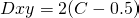. We can thus calculate C from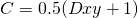.

The first column, index.orig, gives the value of the measure when calculated using the model fitted to the original data and evaluated on the original data (i.e. the naive measure). The second column, training, gives the mean (across the bootstrap samples) of what we defined earlier as. The third column, test, gives the mean of what we defined as, that is applying the models fitted to the bootstrap datasets when evaluated on the original data. As expected, the average of this (test) quantity is lower than the average of the apparent accuracy (training). The difference between these two is then calculated as the estimate of optimism, which here was 0.0914 for Dxy. The optimism correct estimate of Dxy is therefore 0.2533-0.0914=0.1620.

To obtain the optimism corrected value of the c-statistic, we use the earlier stated formula: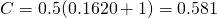. This contrasts with the naive c-statistic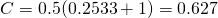.

Let's now repeat the previous code, but increasing the sample size to 1000:

n <- 1000
x <- rnorm(n)
pr <- exp(x)/(1+exp(x))
y <- 1*(runif(n) < pr)
mod <- lrm(y~x, x=TRUE, y=TRUE)
validate(mod, B=1000)
index.orig training   test optimism index.corrected    n
Dxy           0.4558   0.4569 0.4558   0.0011          0.4547 1000
R2            0.2134   0.2153 0.2134   0.0019          0.2115 1000
Intercept     0.0000   0.0000 0.0008  -0.0008          0.0008 1000
Slope         1.0000   1.0000 1.0003  -0.0003          1.0003 1000
Emax          0.0000   0.0000 0.0002   0.0002          0.0002 1000
D             0.1733   0.1752 0.1733   0.0019          0.1714 1000
U            -0.0020  -0.0020 0.0001  -0.0021          0.0001 1000
Q             0.1753   0.1772 0.1732   0.0040          0.1713 1000
B             0.2097   0.2091 0.2101  -0.0010          0.2107 1000
g             1.0817   1.0890 1.0817   0.0073          1.0743 1000
gp            0.2307   0.2311 0.2307   0.0004          0.2303 1000


Now the estimated optimism in Somer's D (Dxy) is just 0.0011, in contrast to the previous figure of 0.0914. This illustrates nicely the fact that optimism in naive measures of predictive accuracy is a function of the size of our dataset - holding other things constant, increasing the sample size reduces the amount of optimism. However, it's important to remember that it's also a function of the complexity of the model we are fitting. To illustrate, let's repeat the simulation one more time, but this time with 50 (normally distributed) covariates. This time we will generate Y independently of the covariates, such that the true model has c-statistic of 0.5, and Somer's Dxy is 0:

n <- 1000
x <- array(rnorm(n*50), dim=c(n,50))
pr <- rep(0.5,n)
y <- 1*(runif(n) < pr)
mod <- lrm(y~x, x=TRUE, y=TRUE)
validate(mod, B=100)
index.orig training    test optimism index.corrected   n
Dxy           0.2565   0.3657  0.1886   0.1771          0.0794 100
R2            0.0687   0.1343  0.0360   0.0983         -0.0297 100
Intercept     0.0000   0.0000  0.0137  -0.0137          0.0137 100
Slope         1.0000   1.0000  0.4752   0.5248          0.4752 100
Emax          0.0000   0.0000  0.1630   0.1630          0.1630 100
D             0.0519   0.1053  0.0264   0.0790         -0.0271 100
U            -0.0020  -0.0020  0.0308  -0.0328          0.0308 100
Q             0.0539   0.1073 -0.0044   0.1117         -0.0578 100
B             0.2371   0.2243  0.2496  -0.0253          0.2624 100
g             0.5400   0.7964  0.3797   0.4167          0.1233 100
gp            0.1290   0.1813  0.0927   0.0885          0.0405 100


Now, despite the dataset being of size 1000, because there are many more covariates, the problem of optimism returns - the optimism in Somer's Dxy is estimated as 0.1771, which translates into optimism in the c-statistic of 0.0886.

For more on the topics of optimism and overfitting, two books I'd recommend are Frank Harrell's Regression Modeling Strategies and Steyerberg's Clinical Prediction Models. For more details on a different bootstrap approach, see chapter 7 of "Elements of Statistical Learning" (free download from the authors' website here).

### 11 thoughts on “Adjusting for optimism/overfitting in measures of predictive ability using bootstrapping”

1.“A simple technique is to split the sample into two parts, using one part to fit the model, and the remainder to assess predictive ability. The problem with this approach is that it is inefficient, since the model is only fitted to a subset of the available data. ” How is this any less efficient than bootstrapping? by sampling with replacement you are also only effectively fitting on a subset of the available data since many data points will be repeated.

•The first approach involves fitting your model using n/2 individuals, where n is your total sample size. With the bootstrapping approach, you fit your model using the full n, and then just use bootstrapping to adjust the naive estimate of model accuracy. So the actual model coefficients estimates from the second approach are more precise than those from the model fitted to just n/2 individuals.

2.Is there a way of obtaining 95% confidence intervals for the bias-corrected estimate of the c statistic?

•Hi. Did you get any answer on this?

3.@Arthur Caye : you can derive 95% CI on the optimism-corrected C by finding the 2.5th and 97.5th percentile of the bootstrap distribution of (C_app – Ob), where Ob is the optimism calculated from the b-th bootstrap sample. Note also, that the average of (C_app – Ob) over all bootstrap samples is exactly equal to the optimism-corrected estimate of C

•Is there a way of extracting the optimism-corrected C or the C_ob from the validate function in the rms package?

4.Hi,

First of all thank you for the nice post. I tried your method and a similar method (shown below). I got better average AUC with the later. I would appreciate if you could provide your opinion. The approach I used is as follows;

I had about 50 predictor variables to start with. First, predictor variables were selected with backward-stepwise algorithm using the original data with 100 bootstraps using ‘bootStepAIC::boot.stepAIC’ R function.

Then, Model fitting and validation was done for 1000 bootstraps of the original data by:

(a) fitting models (with selected predictor variables) to training data (70% of bootstrapped data).
(b) testing the models on test data (30% of bootstrapped data).
(c) calculate Area Under Receiver Operating Curve (AUROC).

Calculate average AUC with 95% CI.

Do you thing the data split is unnecessary? Does it make model fit weaker? I am inclined to this approach because I git better average AUC.

best wishes,

5.Great explanation, thanks.

6.